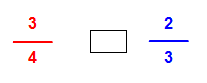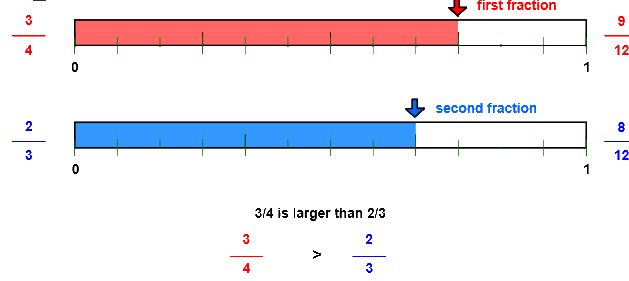# Compare fractions with number line

## Number line models show show how to compare unlike fractions using a common denominator.

COMPARE FRACTIONS
CORRECT:
ATTEMPTS:
SCORE:
PERCENT

# COMPARE FRACTIONS WITH NUMBER LINE MODELS INSTRUCTIONS

Follow the directions in the dialog box after pressing the <START> button. The <EXPLAIN> button may be pressed to see how to do the example.

When Compare Fractions starts,  you will be given two fractions to compare, as in the example below:You are to decide if the fraction on the left is less than, equal to, or greater than the fraction on the right. You will choose < for less than, = for equal to, or > for greater than. Shown in the dialog box are your choices of <, >, or =.

If the denominators are the same, the fraction with the larger numerator is larger and if the numerators are the same, the fraction with the larger denominator is smaller.

The following cmpare fractions illustration was made by Compare Fractions With Lines Designer:

The fractions 34 and 23 are pictured with number lines below:Let's make the denominators the same so that we can compare the numerators. Fractions with the same denominators are like fractions.

Here, we will introduce the idea of the least common denominator or LCDLCD is an idea that will be used in comparing, adding, and subtracting fractions. The LCD is the smallest number that both 4 and 3 will divide into evenly. The LCD for the fractions 34 and 23 is 12 because both denominators 4 and 3 divide evenly into 12.

Then, write each fraction with the common denominator 12 to make them like. The illustration shows that 34 is equal to 912 and 23 is equal to 8/12. Once each fraction is renamed with a common denominator, you can compare the numerators - the larger the numerator the larger the fraction.

Since 34 is greater than 23, you will select the > symbol.

See the program  RENAME IN HIGHER TERMS for more information on renaming fractions.

One way to find the LCD is to see if the smaller denominator 3 will divide evenly into the larger denominator 4.  If not, multiply the larger denominator 4 by 2 to get 8.  Will the smaller denominator 3 divide into 8? No, so multiply the larger denominator by 3 to get 12. Will 3 divide evenly into 12? Yes, so 12 is the LCD for the denominators 3 and 4. If not, then multiply by 4, then 5, etc. until the smaller denominator divides into the product.

Also, thinking of the pictures of the fractions will help you decide which is the larger.

Choose the < (less than) button if you think the first fraction is smaller than the second. Choose the = (equal button) if you think the two fractions are the same size. Choose the > (greater than) if you think the fraction is larger. If correct, number lines showing the comparative sizes of the two fractions will appear. Press the <EXPLAIN> button to see each fraction with the common denominator.

For more instruction on comparng fractions go to How To Compare Fractions.

After you press the <, =, or > button you may press the <REPORT> button. The report will ask for your name but you may submit a code for your name. This report will give same results as on the dialog box. The report may be printed or e-mailed.